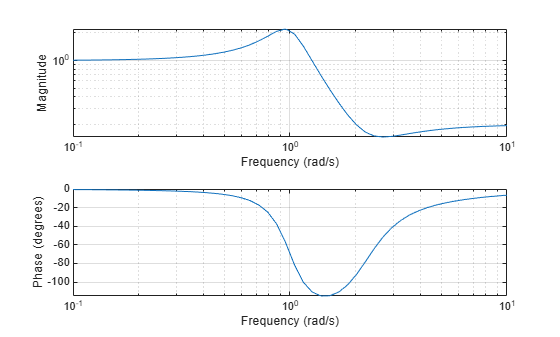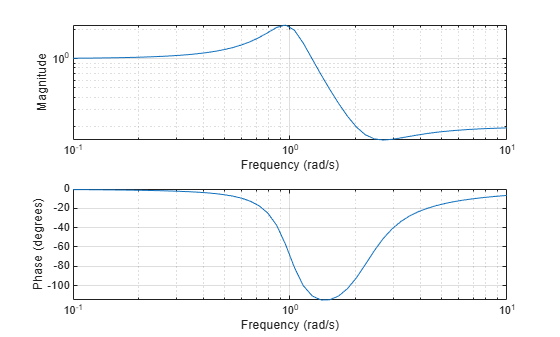freqs

Frequency response of analog filters

Description

example

h = freqs(b,a,w) returns the complex frequency response of the analog filter specified by the coefficient vectors b and a, evaluated at the angular frequencies w.

[h,wout] = freqs(b,a,n) uses n frequency points to compute h and returns the corresponding angular frequencies in wout.

example

freqs(___) with no output arguments plots the magnitude and phase responses as functions of angular frequency in the current figure window. You can use this syntax with either of the previous input syntaxes.

Examples

collapse all

Find and graph the frequency response of the transfer function

$H\left(s\right)=\frac{0.2{s}^{2}+0.3s+1}{{s}^{2}+0.4s+1}.$

a = [1 0.4 1];
b = [0.2 0.3 1];
w = logspace(-1,1);

h = freqs(b,a,w);
mag = abs(h);
phase = angle(h);
phasedeg = phase*180/pi;

subplot(2,1,1)
loglog(w,mag)
grid on
ylabel('Magnitude')

subplot(2,1,2)
semilogx(w,phasedeg)
grid on
ylabel('Phase (degrees)')You can also generate the plots by calling freqs with no output arguments.

figure
freqs(b,a,w)Design a 5th-order analog lowpass Bessel filter with an approximately constant group delay up to $1{0}^{4}$ rad/s. Plot the frequency response of the filter using freqs.

[b,a] = besself(5,10000);   % Bessel analog filter design
freqs(b,a)                  % Plot frequency responseInput Arguments

collapse all

Transfer function coefficients, specified as vectors.

Example: [b,a] = butter(5,50,'s') specifies a fifth-order Butterworth filter with a cutoff frequency of 50 rad/second.

Data Types: single | double

Angular frequencies, specified as a positive real vector expressed in rad/second.

Example: 2*pi*logspace(6,9) specifies 50 logarithmically spaced angular frequencies from 1 MHz (2π × 106 rad/second) and 1 GHz (2π × 109 rad/second).

Data Types: single | double

Number of evaluation points, specified as a positive integer scalar.

Data Types: single | double

Output Arguments

collapse all

Frequency response, returned as a vector.

Angular frequencies at which h is computed, returned as a vector.

Algorithms

freqs returns the complex frequency response of an analog filter specified by b and a. The function evaluates the ratio of Laplace transform polynomials

$H\left(s\right)=\frac{B\left(s\right)}{A\left(s\right)}=\frac{b\left(1\right){s}^{n}+b\left(2\right){s}^{n-1}+\cdots +b\left(n+1\right)}{a\left(1\right){s}^{m}+a\left(2\right){s}^{m-1}+\cdots +a\left(m+1\right)}$

along the imaginary axis at the frequency points s = :

s = 1j*w;
h = polyval(b,s)./polyval(a,s);